Breaking News
Home / SSCE / Tricks To Answer Neco Further Mathematics And Hot Questions(What Neco Sets)

Tricks To Answer Neco Further Mathematics And Hot Questions(What Neco Sets)2020 NECO FURTHER Mathematics: What are the areas NECO will set Further Mathematics Questions from and how do I get NECO F Maths 2020 OBJ And Essay Questions? Neco 2020 Further Maths Questions is what we shall discuss here today.

NECO Further Mathematics is not compulsory for every Neco 2020 science Candidate. Read on for Sample Questions Neco would set in 2020 Further Maths or click here for Neco 2020 Further Mathematics syllabus.

NECO 2020 Further Mathematics Questions And Answers

You have asked the questions, what are the areas Neco sets questions in further mathematics? I am here to give you the bold answers to your question.

To make this topic easy for you to understand, I will divide it into three sections. The three sections are:

1. NECO 2020 Further Maths Objective Questions
2. 2020 Neco Further Mathematics Essay Questions And Answers.
3. Instructions To Pass Neco 2020 Examination.

NECO 2020 Further Maths Objective Questions

As usual, you will be given questions and options A to E to choose from. Normally, the number of objective questions (OBJ) you are to answer in Neco 2020 Further mathematics is 50.

The Following Are Sample Neco Objective Questions on further mathematics.

Note: Last Neco Further mathematics Examination date for Objective and Essay paper was on the 28th of June 2018.

Further Mathematics Neco 2020 Theory Questions And Answers

The following are the kind of questions you should expect in neco 2020 Further Maths Theory or Essay. They are hot cake questions:

Questions 1

Points (2, 1) and (6, 7) are opposite vertices of a square which is inscribed in a circle.
Find the:

1. centre of the circle;
2. equation of the circle.

Question 2

If f’’(x) = 2, f’(1) = 0 and f(0) = -8, find f(x).

Question 5

A car moving with an initial velocity, u, travels in a straight line with a constant acceleration of 3 ms-2 until it attains a velocity of 33 ms-1 after 6 seconds. Calculate the distance travelled by the car.

Question 6

Forces of magnitude 3N, 4N and 2N act along the vectors j, –i + j and i + j respectively. Calculate, correct to one decimal place, the magnitude of the resultant of the forces.

Question 7

1. The functions f: x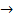x2 + 1 and g: x5 – 3x are defined on the set of real numbers, R.
2. State the domain of f-1, the inverse of f.
3. Find g-1(2).

Evaluate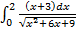Question 8

1. If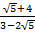–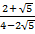= a + b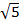, find the values of a and b.
2. (i) Evaluate: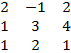.

(ii) Using the result in 10(b)(i), find, correct to two decimal places, the value of x in the system of equations.
2x – y + 2z + 5 = 0
x + 3y + 4z – 1 = 0
x + 2y + z + 2 = 0

Neco 2020 Examination Instructions

1. Do not open your question paper until you are told to do so
2. USE HB pencil throughout in the OBJ Section
3. You are free to use Biro in the theory part
4. You are allowed to use calculator to solve
5. Make sure that you fill your name, Subject, paper, write WASSCE, paper code and other examination details where necessary.
6. Ensure that the texts in your question papers are boldly printed
7. Behave yourself.
8. Don’t let invigilators catch you using expo

Related To 2020 Further Math Essay & OBJ:

Neco 2020 Essay and obj questions
Flashlearners further math answer neco 2020
www.neco 2020 further mathematics
Further mathematics obj neco 2020
neco exam timetable
2020 neco further maths questions
OBJ and essay further mathematics 2020
Essay and obj expo answers for mathematics further
2020 neco further maths syllabus

That’s all for now… I shall update you when more real live questions and answers come up. However, I advise that you are hard working so as to pass your Neco once and for all.